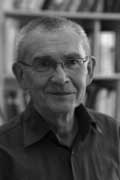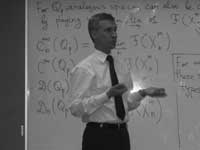All esoteric subjects have their own secret (sacred) texts. If you opened the Da Vinci Code (or even better, the original The Holy blood and the Holy grail) you will known about a mysterious collection of documents, known as the “Dossiers secrets“, deposited in the Bibliothèque nationale de France on 27 April 1967, which is rumoured to contain the mysteries of the Priory of Sion, a secret society founded in the middle ages and still active today…

The followers of F-un, for $\mathbb{F}_1$ the field of one element, have their own collection of semi-secret texts, surrounded by whispers, of which they try to decode every single line in search of enlightenment. Fortunately, you do not have to search the shelves of the Bibliotheque National in Paris, but the depths of the internet to find them as huge, bandwidth-unfriendly, scanned documents.The first are the lecture notes “Lectures on zeta functions and motives” by Yuri I. Manin of a course given in 1991.

One can download a scanned version of the paper from the homepage of Katia Consani as a huge 23.1 Mb file. Of F-un relevance is the first section “Absolute Motives?” in which

“…we describe a highly speculative picture of analogies between arithmetics over $\mathbb{F}_q$ and over $\mathbb{Z}$, cast in the language reminiscent of Grothendieck’s motives. We postulate the existence of a category with tensor product $\times$ whose objects correspond not only to the divisors of the Hasse-Weil zeta functions of schemes over $\mathbb{Z}$, but also to Kurokawa’s tensor divisors. This neatly leads to teh introduction of an “absolute Tate motive” $\mathbb{T}$, whose zeta function is $\frac{s-1}{2\pi}$, and whose zeroth power is “the absolute point” which is teh base for Kurokawa’s direct products. We add some speculations about the role of $\mathbb{T}$ in the “algebraic geometry over a one-element field”, and in clarifying the structure of the gamma factors at infinity.” (loc.cit. p 1-2)

I’d welcome links to material explaining this section to people knowing no motives.The second one is the unpublished paper “Cohomology determinants and reciprocity laws : number field case” by Mikhail Kapranov and A. Smirnov.

This paper features in blog-posts at the Arcadian Functor, in John Baez’ Weekly Finds and in yesterday’s post at Noncommutative Geometry.

You can download every single page (of 15) as a separate file from here. But, in order to help spreading the Fun-gospel, I’ve made these scans into a single PDF-file which you can download as a 2.6 Mb PDF. In the introduction they say :

“First of all, it is an old idea to interpret combinatorics of finite sets as the $q \rightarrow 1$ limit of linear algebra over the finite field $\mathbb{F}_q$. This had lead to frequent consideration of the folklore object $\mathbb{F}_1$, the “field with one element”, whose vector spaces are just sets. One can postulate, of course, that $\mathbf{spec}(\mathbb{F}_1)$ is the absolute point, but the real problem is to develop non-trivial consequences of this point of view.”

They manage to deduce higher reciprocity laws in class field theory within the theory of $\mathbb{F}_1$ and its field extensions $\mathbb{F}_{1^n}$. But first, let us explain how they define linear algebra over these absolute fields.

Here is a first principle : in doing linear algebra over these fields, there is no additive structure but only scalar multiplication by field elements. So, what are vector spaces over the field with one element? Well, as scalar multiplication with 1 is just the identity map, we have that a vector space is just a set. Linear maps are just set-maps and in particular, a linear isomorphism of a vector space onto itself is a permutation of the set. That is, linear algebra over $\mathbb{F}_1$ is the same as combinatorics of (finite) sets.

A vector space over $\mathbb{F}_1$ is just a set; the dimension of such a vector space is the cardinality of the set. The general linear group $GL_n(\mathbb{F}_1)$ is the symmetric group $S_n$, the identification via permutation matrices (having exactly one 1 in every row and column)

Some people prefer to view an $\mathbb{F}_1$ vector space as a pointed set, the special element being the ‘origin’ $0$ but as $\mathbb{F}_1$ doesnt have a zero, there is also no zero-vector. Still, in later applications (such as defining exact sequences and quotient spaces) it is helpful to have an origin. So, let us denote for any set $S$ by $S^{\bullet} = S \cup { 0 }$. Clearly, linear maps between such ‘extended’ spaces must be maps of pointed sets, that is, sending $0 \rightarrow 0$.

The field with one element $\mathbb{F}_1$ has a field extension of degree n for any natural number n which we denote by $\mathbb{F}_{1^n}$ and using the above notation we will define this field as :

$\mathbb{F}_{1^n} = \mu_n^{\bullet}$ with $\mu_n$ the group of all n-th roots of unity. Note that if we choose a primitive n-th root $\epsilon_n$, then $\mu_n \simeq C_n$ is the cyclic group of order n.

Now what is a vector space over $\mathbb{F}_{1^n}$? Recall that we only demand units of the field to act by scalar multiplication, so each ‘vector’ $\vec{v}$ determines an n-set of linear dependent vectors $\epsilon_n^i \vec{v}$. In other words, any $\mathbb{F}_{1^n}$-vector space is of the form $V^{\bullet}$ with $V$ a set of which the group $\mu_n$ acts freely. Hence, $V$ has $N=d.n$ elements and there are exactly $d$ orbits for the action of $\mu_n$ by scalar multiplication. We call $d$ the dimension of the vectorspace and a basis consists in choosing one representant for every orbits. That is, $~B = { b_1,\ldots,b_d }$ is a basis if (and only if) $V = { \epsilon_n^j b_i~:~1 \leq i \leq d, 1 \leq j \leq n }$.

So, vectorspaces are free $\mu_n$-sets and hence linear maps $V^{\bullet} \rightarrow W^{\bullet}$ is a $\mu_n$-map $V \rightarrow W$. In particular, a linear isomorphism of $V$, that is an element of $GL_d(\mathbb{F}_{1^n})$ is a $\mu_n$ bijection sending any basis element $b_i \rightarrow \epsilon_n^{j(i)} b_{\sigma(i)}$ for a permutation $\sigma \in S_d$.

An $\mathbb{F}_{1^n}$-vectorspace $V^{\bullet}$ is a free $\mu_n$-set $V$ of $N=n.d$ elements. The dimension $dim_{\mathbb{F}_{1^n}}(V^{\bullet}) = d$ and the general linear group $GL_d(\mathbb{F}_{1^n})$ is the wreath product of $S_d$ with $\mu_n^{\times d}$, the identification as matrices with exactly one non-zero entry (being an n-th root of unity) in every row and every column.

This may appear as a rather sterile theory, so let us give an extremely important example, which will lead us to our second principle for developing absolute linear algebra.

Let $q=p^k$ be a prime power and let $\mathbb{F}_q$ be the finite field with $q$ elements. Assume that $q \cong 1~mod(n)$. It is well known that the group of units $\mathbb{F}_q^{\ast}$ is cyclic of order $q-1$ so by the assumption we can identify $\mu_n$ with a subgroup of $\mathbb{F}_q^{\ast}$.

Then, $\mathbb{F}_q = (\mathbb{F}_q^{\ast})^{\bullet}$ is an $\mathbb{F}_{1^n}$-vectorspace of dimension $d=\frac{q-1}{n}$. In other words, $\mathbb{F}_q$ is an $\mathbb{F}_{1^n}$-algebra. But then, any ordinary $\mathbb{F}_q$-vectorspace of dimension $e$ becomes (via restriction of scalars) an $\mathbb{F}_{1^n}$-vector space of dimension $\frac{e(q-1)}{n}$.

Next time we will introduce more linear algebra definitions (including determinants, exact sequences, direct sums and tensor products) in the realm the absolute fields $\mathbb{F}_{1^n}$ and remarkt that we have to alter the known definitions as we can only use the scalar-multiplication. To guide us, we have the second principle : all traditional results of linear algebra over $\mathbb{F}_q$ must be recovered from the new definitions under the vector-space identification $\mathbb{F}_q = (\mathbb{F}_q^{\ast})^{\bullet} = \mathbb{F}_{1^n}$ when $n=q-1$. (to be continued)

Published in absolute

## One Comment

This site uses Akismet to reduce spam. Learn how your comment data is processed.